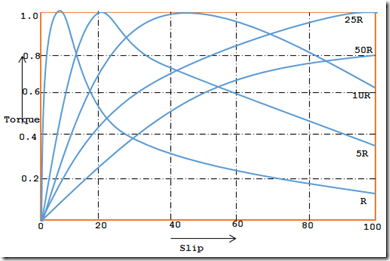# Slip Torque Characteristics of an Induction Motor

### Stable and Unstable Region of the Slip Torque Characteristics:The torque, T of a three phase induction motor is given by
T=(KΦs E2R2) / (R2+(sX2)2)
Where,
Φ = flux
S = slip
E2 = Rotor induced EFM per Phase at standstill
R2 = Rotor resistance per phase
X2 = Rotor reactance per phase at standstill
K = a constantThe slip torque curves for various ranges of slip with different R2 are drawn. For slip values ranging from 0 to 1 these curves are drawn.
Using the above relation, the following cases are considered:

Case-1:
From the above relation when s=0, then the torque, T is also zero. Therefore, the curve starts from (0, 0).

Case-2:
When the speed of the rotor is near about the synchronous speed, then the slip s value is very small. Therefore the term (s.X2) is small. On comparing this value with R2, the value (s.X2) is negligible. Substituting these values in the torque equation, we get,
T = (KΦsE2R2) / R22
T ∝ s / R2Since Φ, E2 are constants.
Or, T∝s, if R2 is constant. From this, it is understood that for low values of slip the torque slip, curve is more or less a straight line.
Case-3:
When the load on the motor increases, the rotor speed falls down. Then the slip value increases. From the above relation it is seen that, when slip s increases the torque, T also increases. The torque will attain a maximum value, at s = (R2/X2). This torque is called pull out or break down or stalling torque. Further increases in load on the motor causes, the slip to increase still more. For higher values of slip, R2 is negligible as compare to (sX2). Then the torque equation becomes,

T∝ {(KΦsE2)/(sX2)2’}
T∝ {s/(sX2)2’}, since Φ & E2 are constant.
T∝ 1/s i.e. X2 is constant

Therefore, for higher values of slip, the torque slip curve is a rectangular hyperbola.

For various values of R, the family of torque slip curve is as shown in the figure 1. From these curves, it is clear that, beyond the point of maximum torque, the increased load on the motor results in a decrease in torque developed. Any further more increase in load on the motor results in the slowing down of motor and it finally stops. The speed of the motor comes to zero, when the slip reaches to 1. (if the load on the motor is too much, the rotor speed comes to zero and it stops, then slip s = (Ns – 0) / Ns and equal to 1).

Hence the stable operating region of the motor lies for the slip values, s=0, and that corresponds to maximum torque. The operating region is indicating hatched in the figure 2.1 CommentsComments

#### Read Comment Policy We have Zero Tolerance to Spam. Chessy Comments and Comments with Links will be deleted immediately upon our review.

1.Try not to get restless
If you imagine that you essentially play fluctuated Satta numbers and may expand the conceivable outcomes of winning. Then, at that point, it's valid, but doing it for partner unnecessary time inside the Satta King 786 will make you lose the game. Betting is that of course any place you wish to get a handle on Satta results in 786 spots in some genuine money. Accordingly, you should be clear with no misconception once you are making a bet.